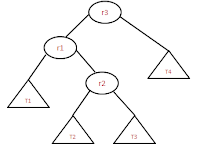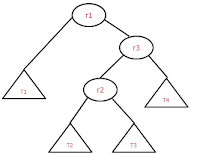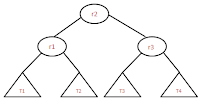## Friday, August 24, 2012

### AVL tree double rotation!!

Double rotation: need to do two rotations (left-right or right-left) to balance the tree. In this there are two types of sub rotations
• Right Left rotation
• Left Right rotation

﻿Before Rotation
﻿ Right-Left rotation:  This is double rotation method in which we need to do two rotatios left and right. And this method needs to apply if the tree is as shown in the below image.
• Here r3 is un balanced and we need to do RL rotation because r2 is right child of r1 and r1 is left child of un balanced r3.
• ﻿﻿﻿﻿
• Rotation is done in such a way that T1<r1<T2<r2<T3<r3<T4 property should satisfy after rotation.
•After Rotation
• To balance the tree, make r2 as root node and follow the binary search tree property for all other nodes.
• Sample C code for Right Left rotation is given below. ﻿﻿
```
//below steps are making the tree as shown in the image before rotation
r1 = r3-<left;
r2 = r1-<right;
t2 = r2-<left;
t3 = r2-<right;

// actaul rotatiosn happens here
r1-<right = t2;
r3-<left = t3;
r2-<left = r1;
r2-<right = r3;

//updte the new heihts for r1, r2, r3
set_height(r1);
set_height(r2);
set_height(r3);

*r = r2;

```
Left Right rotation: This is double rotation method in which we need to do two rotatios right and left. And this method needs to apply if the tree is as shown in the below image.
﻿Before Rotation
﻿
• Here r1 is un balanced and we need to do RL rotation because r2 is left child of r3 and r3 is right child of un balanced r1.
• Rotation is done in such a way that T1<r1<T2<r2<T3<r3<T4 property should satisfy after rotation.
•After Rotation
• To balance the tree, make r2 as root node and follow the binary search tree property for all other nodes.
• Sample C code for Right Left rotation is given below.
```    //below steps are making the tree as shown in the image before rotation
r3 = r1-<right;
r2 = r3-<left;
t2 = r2-<left;
t3 = r2-<right;

// actaul rotatiosn happens here
r1-<right = t2;
r3-<left = t3;
r2-<left = r1;
r2-<right = r3;

//updte the new heihts for r1, r2, r3
set_height(r1);
set_height(r2);
set_height(r3);

*r = r2;

```